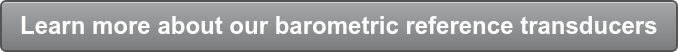One of the frequent questions Mensor customers ask is what additional uncertainty is added to their calibration when measuring pressure in emulation mode.

## What is Emulation Mode?

Emulation mode is when a barometric reference transducer is added to an instrument and allows the use of absolute transducers to emulate gauge pressures or gauge transducers to emulate absolute pressures. If using absolute transducers, the instrument subtracts the barometric pressure to emulate gauge pressure. Since they are both tracking atmospheric pressures, they track each other. If using gauge transducers, the instrument adds the barometric pressure from the barometer to emulate absolute pressure.Many people think they simply have to add the uncertainty of the barometer to the uncertainty of the main transducer to get a combined uncertainty, but that is not the case.  The purpose of this blog is to explain in detail the methodology used to calculate the uncertainty in an emulation mode.

## Example #1 – Absolute transducers emulating gauge pressures

Suppose we have a 125 kPa absolute transducer coupled with a barometer to emulate gauge pressure.
The uncertainty components are:
1.    Uncertainty of the absolute transducer
2.    Drift of the barometer during the time of the calibration/test being performed
3.    Resolution of the barometric reading

Note: The absolute “accuracy” of the barometric reference has nothing to do with it – IF the absolute transducer is zeroed in gauge emulation mode.

Let’s assume the following :
1.    Uncertainty of the absolute transducer: 0.01% FS of 125 kPa = 0.0125 kPa (k = 2 so the component uncertainty is 0.0125/2 = 0.00625 kPa)
2.    If the test takes 2 hours, and the drift of the transducer is 0.01% R/year then the drift during the 2 hour test is: 125 kPA*0.01%/365 days/24 hr/day*2 hr = ~0.0000028 kPa
3.    The resolution of the barometer (in kPa) = 0.001 kPa

To include all of the components for the total uncertainty (per the GUM: Guide to the Expression of Uncertainty in Measurement), it would be the root sum squared (RSS) of #1, #2 and #3 (#3 must be divided by sqrt(3)) – this is a statistical convention for rectangular distributions: 0.001/SQRT(3)=0.000577) multiplied by 2 to get a ~95% confidence level.

So the equation for the Total Uncertainty is:

### Sqrt(0.00625^2 + 0.0000028^2 + 0.000577^2)*2 = 0.0125532 kPa

So, the additional error in kPa in emulation mode is the total uncertainty calculated above, minus the uncertainty of the absolute transducer. This is 0.0125532 – 0.0125  = 0.0000532 kPa or 0.01004%, which is less than the resolution of the instrument.  Of course, the additional uncertainty gets even less significant if the pressure range of the absolute transducer increases.

## Example #2 – Gauge transducers emulating absolute pressures

This is a little trickier because it is more difficult to zero absolute transducers. If you can, it is exactly the same as above except for the Note listed – the drift error of the barometer must be considered in this case. The combined accuracy is also dependent on the range of the primary transducer.

This time let’s assume we have a gauge transducer ranged from -1 bar to +1 bar, a span of 2 bar, and we want to emulate 0-2 bar absolute, using the same process as the example above:
1.    0.01% * 2 bar = 0.0002 bar (k=2 so the component uncertainty is .0002 bar/2= 0.0001 bar)
2.    Again, if the test takes 2 hours, and the drift of the native transducer is 0.01% R/year then two hours of drift would be 2 bar*.0001/365 days/24 hr/day*2 hr 0.00000004 bar
3.    The resolution of the barometer is = 0.00001 bar
4.    The worst case drift error of the barometer at 1 bar is: 1 bar * 0.01% = 0.0001 bar (k=2 so the component uncertainty is 0.0001 bar/2 = 0.00005 bar)

To include all of the components for the total uncertainty (per the GUM), it would be the root sum squared (RSS) of #1, #2 and #3 (#3 must be divided by sqrt(3)) – this is a statistical convention for  rectangular distributions: 0.00001/SQRT(3)=0.000006), and #4, then multiplied by 2 to get a ~95% confidence level.

RSS them together and we get:

### Sqrt(0.0001^2 + 0.00000004 ^2 + 0.000006^2+0.00005^2)*2 = 0.000224 bar

This gives a total uncertainty of 0.000224 bar or an increase due to emulation of 0.000224 - 0.0002 = 0.000024 bar – which only 0.0012% FS.

If you do the same thing on a -1 to 100 bar gauge transducer, the nominal uncertainty is 0.0101 bar, emulation uncertainty is 0.0101005 and again, the additional error is 0.0000005 bar, which is 0.0000005% FS and is insignificant.